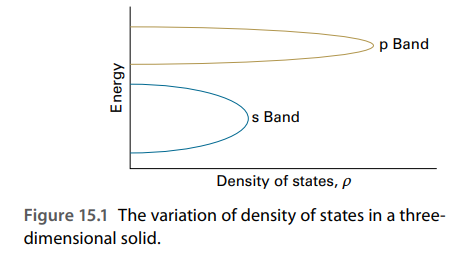×
Get Full Access to Atkins' Physical Chemistry - 11 Edition - Chapter 15c - Problem P15c.6
Get Full Access to Atkins' Physical Chemistry - 11 Edition - Chapter 15c - Problem P15c.6

×ISBN: 9780198769866 2042

## Solution for problem P15C.6 Chapter 15C

Atkins' Physical Chemistry | 11th Edition

• Textbook Solutions
• 2901 Step-by-step solutions solved by professors and subject experts
• Get 24/7 help from StudySoup virtual teaching assistantsAtkins' Physical Chemistry | 11th Edition

4 5 1 341 Reviews
26
3
Problem P15C.6

The energy levels of N atoms in the tight-binding Hückel approximation are the roots of a tridiagonal determinant (eqn 15C.1):

$$E_{k}=\alpha+2 \beta \cos \frac{k \pi}{N+1}$$ \quad $$k=1,2, \ldots, N$$If the atoms are arranged in a ring, the solutions are the roots of a ‘cyclic’ determinant:

$$E_{k}=\alpha+2 \beta \cos \frac{2 k \pi}{N}$$ \quad $$k=0, \pm 1, \pm 2, \ldots, \pm \frac{1}{2} N$$

(for N even). Discuss the consequences, if any, of joining the ends of an initially straight length of material.

Text Transcription:

E_k=alpha+2 beta cos k pi/N+1

k=1,2, ldots, N

E_k=alpha+2 beta cos 2k pi/N

k=0, pm 1, pm 2, ldots, pm1/2 N

Step-by-Step Solution:
Step 1 of 3

Chemistry 107­001 (M,T,W,TH) Dr. Mentele Week 1: 2/5 – 2/8 Nomenclature: “the naming game” *remember: ionic = metal + nonmetal; molecular = nonmetal + nonmetal 1. Naming Ionic Compounds: (type I & type II)  Polyatomic ions in a compound i. Cations (+): name stays the same ii. Anion (­): ending in the prefix ­ide Metal Non­Metal Chemical Formula Name Calcium Bromine CaBr 2 calcium bromide Magnesium Oxygen MgO magnesium oxide Aluminum Nitrogen AlN aluminum nitride Ionic Compounds Type I (+1) Type II (+2)  One ion  More than one ion  Al3+, Zn2+, Ag1+  All other metals  Ionic compounds: w/ type II metals o Same rules: cation + anion o Use roman numerals for charge of metal that can have more that one charge  Ex. iron(III)  Fe3+  Ex. iron(II) chloride  Cl 2 o Common names:  Ion with lower charge = “ous”  Ion with higher charge = “ic”  Ex. iron(II) chloride = ferrous chloride  Ex. iron(II

Step 2 of 3

Step 3 of 3

## Discover and learn what students are asking

Statistics: Informed Decisions Using Data : The Design of Experiments
?Explain the difference between a single-blind and a double- blind experiment.

Statistics: Informed Decisions Using Data : Applications of the Normal Distribution
?In Problems 19–22, find the value of ?? ?0.025

Statistics: Informed Decisions Using Data : The Randomized Complete Block Design
?Given the following ANOVA output, answer the questions that follow: (a) The researcher wants to test H0: m1 = m2 = m3 =

Statistics: Informed Decisions Using Data : The Randomized Complete Block Design
?How does the completely randomized design differ from a randomized complete block design?

Unlock Textbook Solution